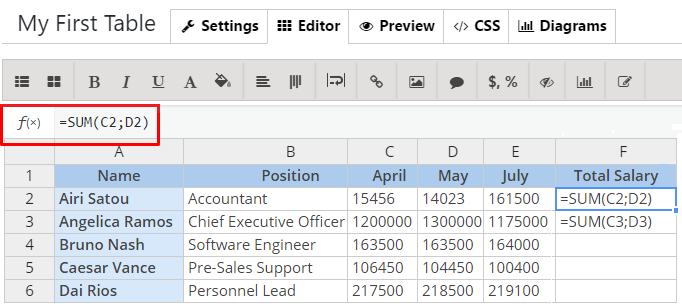﻿ How to use formulas in tables? WP Table Editor by Supsystic

# Use Formulas in Tables on Ease

To make a formula you need to do next steps:

1. Open «Editor» tab in the table settings.
2. Select a cell and type an equal sign «=» into f(x) field above the table to start the formula.3. Complete the formula by one of the accessible ways:
• Combination of numbers and operators.Example: `=3+7`
• Names of cells inserting an operator between them.Example: `=A1+B1+C1`
• Choose one of the standard formulas with cells’ names inside the parentheses «()» and separators between them.Example: `=SUM(A1;B1;C1)`
4. Click «Save» button to apply the changes.
Important! You can not see the formula result on the Editor tab – only on Preview tab or on the site page.
Categorias
Latest Articles